Latest Banking jobs   »

# Quantitative Aptitude Quiz For IBPS Clerk Prelims 2022- 15th September

Directions (1-5): Bar graph given below show total number of students in five different schools (A, B, C, D & E) in two different years (2021, 2022). Read the following bar graph carefully and answer the questions given below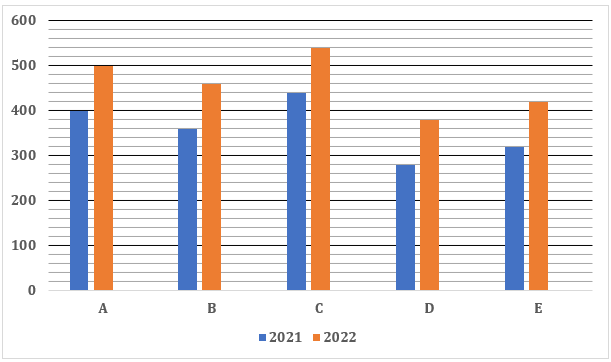Q1. The ratio of number of boys to girls in school B and that in D in 2021 is 11:7 & 9:5 respectively. Find the difference between total number of boys in B & D together and number of total girls in both schools in 2021?
(a) 120
(b) 160
(c) 180
(d) 240
(e) 200

Q2. Find the total number of students in school A in 2022 is what percent (approximately) more or less than total number of students in school C in 2021?
(a) 14%
(b) 18%
(c) 26%
(d) 22%
(e) 8%

Q3. If total number of students in school F in 2022 is 66.66% more than total number of students in E in 2022, then find total number of students in school D and F together in 2022?
(a) 1180
(b) 1140
(c) 1120
(d) 1080
(e) 1160

Q4. Find the ratio of total number of students in E and that in C together in 2022 to total number of students in B and that in D in 2021?
(a) 5:2
(b) 3:7
(c) 3:5
(d) 4:7
(e) 3:2

Q5. Average number of students in A, B & C in 2022 is how much more or less than average number of students in D & E in 2021?
(a) 240
(b) 200
(c) 160
(d) 180
(e) 280

Directions (6-10): Given below is the table which shows the percentage of boys in two school A and B in five different years. Read the data carefully and answer the questions.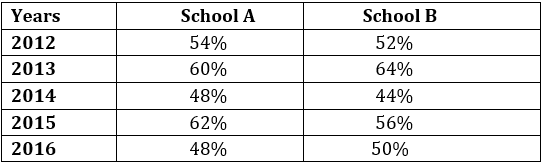Note: Total students in any school = Total boys + Total girls in each school

Q6. In 2012, ratio of boys in school A to school B is 45 : 52 and total students in both school in 2012 is 1100. Find the total number of girls in both school in same year.
(a) 568
(b) 528
(c) 518
(d) 418
(e) 488

Q7. If in 2014, boys in school A and B are 288 and 264 respectively then, find total number of girls in both school in 2014.
(a) 594
(b) 640
(c) 564
(d) 648
(e) 630

Q8. If boys in school A in 2014 and girls in school B in 2012 are equal then boys in school B in 2012 are what percent of girls in school A in 2014.
(a) 85%
(b) 95%
(c) 90%
(d) 80%
(e) 100%

Q9. In 2016, girls in school A are 16⅘% less than girls in school B. Find the ratio of boys in school A to that of school B in 2016.
(a) 100 : 123
(b) 98 : 117
(c) 98 : 125
(d) 92 : 117
(e) 96 : 125

Q10. If total students in school A in 2015 and total student in B in 2013 are 700 and 400 respectively, then find the average number of boys in school A in 2015 and boys in school B in 2013.
(a) 344
(b) 345
(c) 348
(d) 368
(e) 358

Directions (11-15): Read the following line chart carefully and answer the questions given below. Line chart show total number of masks (type A and type B) produced and percentage of number of type A masks produced by a company in five different days of a week.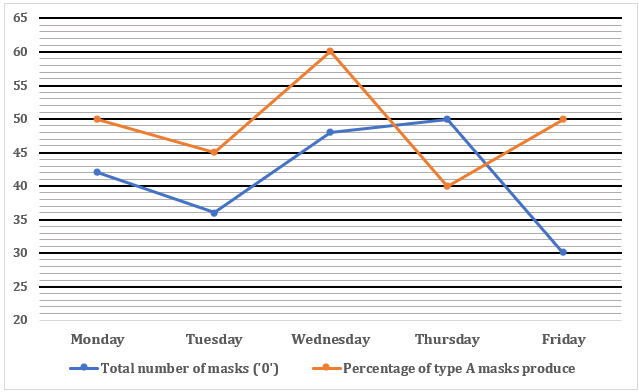Q11. Find the difference between number of type B masks produced on Monday to that on Friday?
(a) 45
(b) 50
(c) 75
(d) 60
(e) 55

Q12. Find the ratio of number of type A masks produced on Tuesday to number of type B masks produced on Thursday?
(a) 27 :55
(b) 29 :50
(c) 27 :50
(d) 21 :59
(e) 22 :57

Q13. Number of type B masks produced on Thursday is what percent more/less than number of type A masks produced on Friday?
(a) 125%
(b) 75%
(c) 50%
(d) 150%
(e) 100%

Q14. If number of type C masks produced on Tuesday is 20% more than number of type A masks produced on Monday, then find the average number of all types of masks produced on Tuesday?
(a) 204
(b) 218
(c) 265
(d) 194
(e) 156

Q15. If type B masks produced on Saturday are 40% less than that on Thursday, then type A masks produced on Wednesday is what percent of type B masks produced on Saturday?
(a) 180%
(b) 160%
(c) 155%
(d) 125%
(e) 100%

Solutions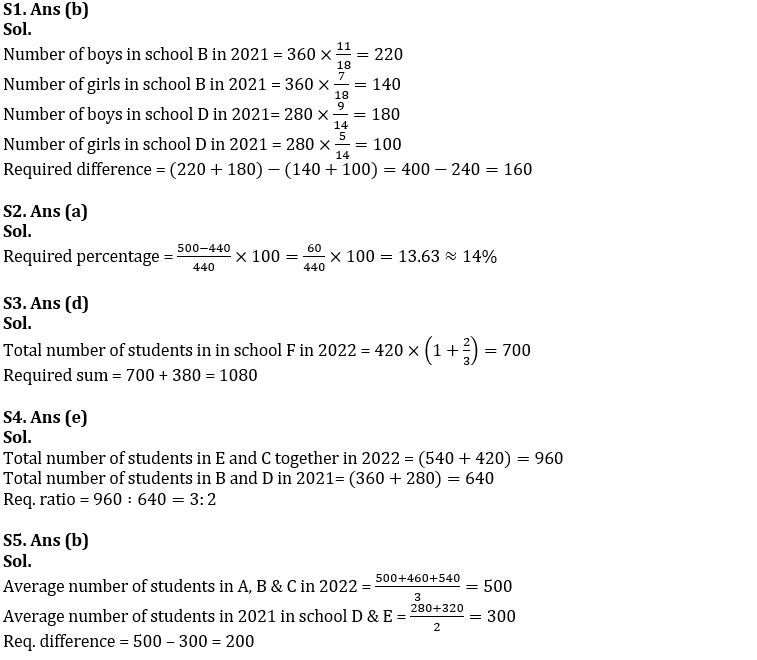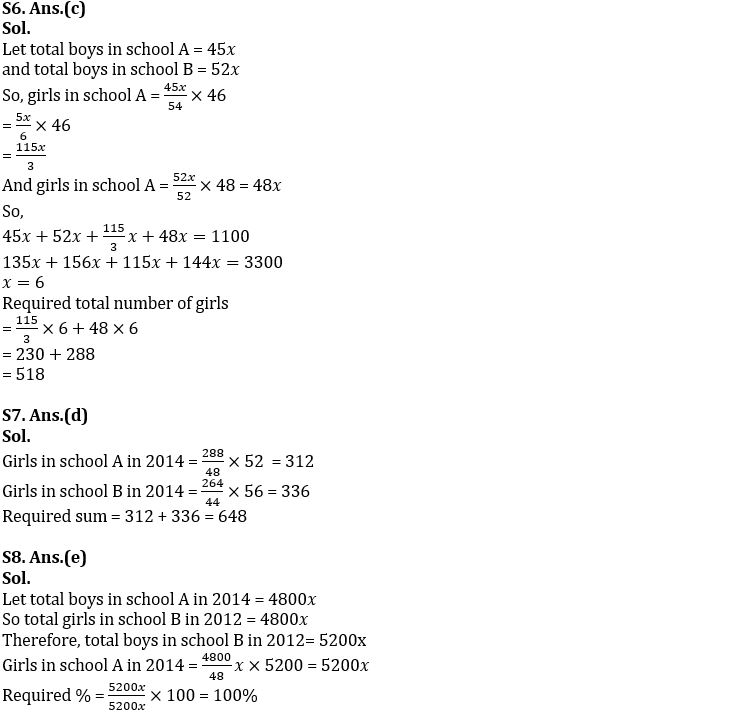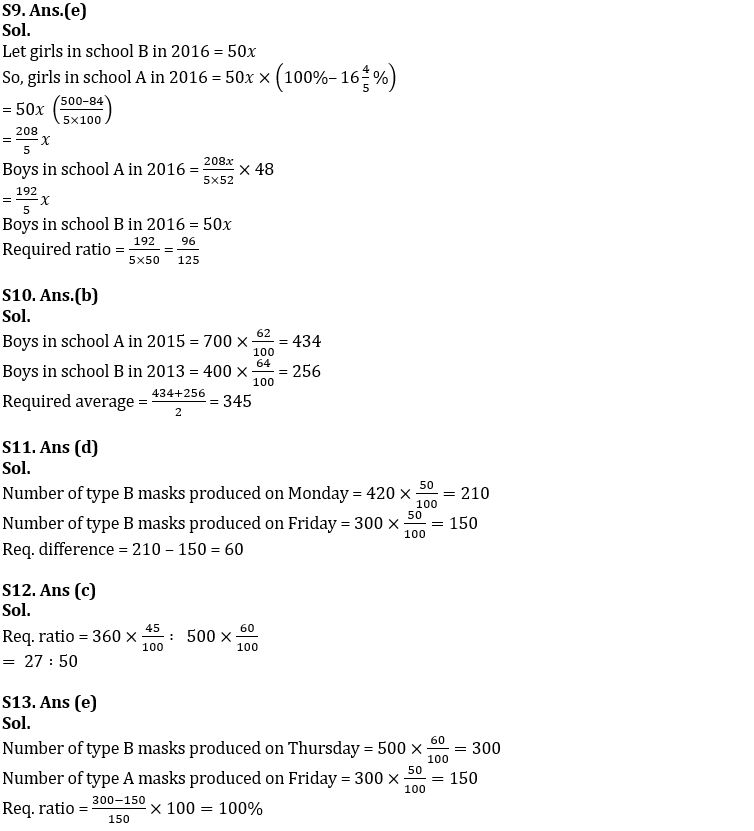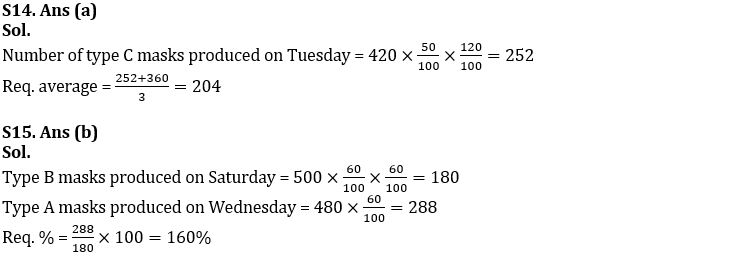#### Congratulations!Download Hindu Review of October 2021: Free PDF